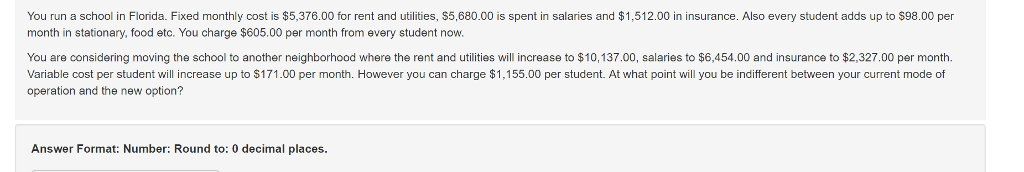# Question & Answer: You run a school in Florida. Fixed monthly cost is \$5,376.00 for rent and utilities, \$5,680.00 is spent in salaries and \$1,512.00 in insu…..You run a school in Florida. Fixed monthly cost is \$5,376.00 for rent and utilities, \$5,680.00 is spent in salaries and \$1,512.00 in insurance. Also every student adds up to \$98.00 per month in stationary, food etc. You charge \$605.00 per month from every student now. You are considering moving the school to another neighborhood where the rent and utilities will increase to \$10,137.00, salaries to \$6,454.00 and insurance to \$2,327.00 per month. Variable cost per student will increase up to \$171.00 per month. However you can charge \$1,155.00 per student. At what point will you be indifferent between your current mode of operation and the new option? Answer Format: Number: Round to: 0 decimal places.

Don't use plagiarized sources. Get Your Custom Essay on
Question & Answer: You run a school in Florida. Fixed monthly cost is \$5,376.00 for rent and utilities, \$5,680.00 is spent in salaries and \$1,512.00 in insu…..
GET AN ESSAY WRITTEN FOR YOU FROM AS LOW AS \$13/PAGE

Let Indifferent number of students be X which mean X is the indifferent point between Current Option and New Option.

Current Option:

Fixed Monthly Expenses = Rent and Utilities + Salaries + Insurance
Fixed Monthly Expenses = \$5,376.00 + \$5,680.00 + \$1,512.00
Fixed Monthly Expenses = \$12,568.00

Variable Expense per student = \$98.00
Monthly Fees per student = \$605.00

Profit from Operation = Fees Received – Total Variable Expense – Total Fixed Monthly Expense
Profit from Operation = \$605.00*X – \$98.00*X – \$12,568.00
Profit from Operation = \$507.00*X – \$12,568.00

New Option:

Fixed Monthly Expenses = Rent and Utilities + Salaries + Insurance
Fixed Monthly Expenses = \$10,137.00 + \$6,454.00 + \$2,327.00
Fixed Monthly Expenses = \$18,918.00

Variable Expense per student = \$171.00
Monthly Fees per student = \$1,155.00

Profit from Operation = Fees Received – Total Variable Expense – Total Fixed Monthly Expense
Profit from Operation = \$1,155.00*X – \$171.00*X – \$18,918.00
Profit from Operation = \$984.00*X – \$18,918.00

Profit from Operation under Current Option = Profit from Operation under New Option
\$507.00*X – \$12,568.00 = \$984.00*X – \$18,918.00
\$6,350 = \$477*X
X = 14 students

So, Current Option and New Option will be indifferent at 14 students